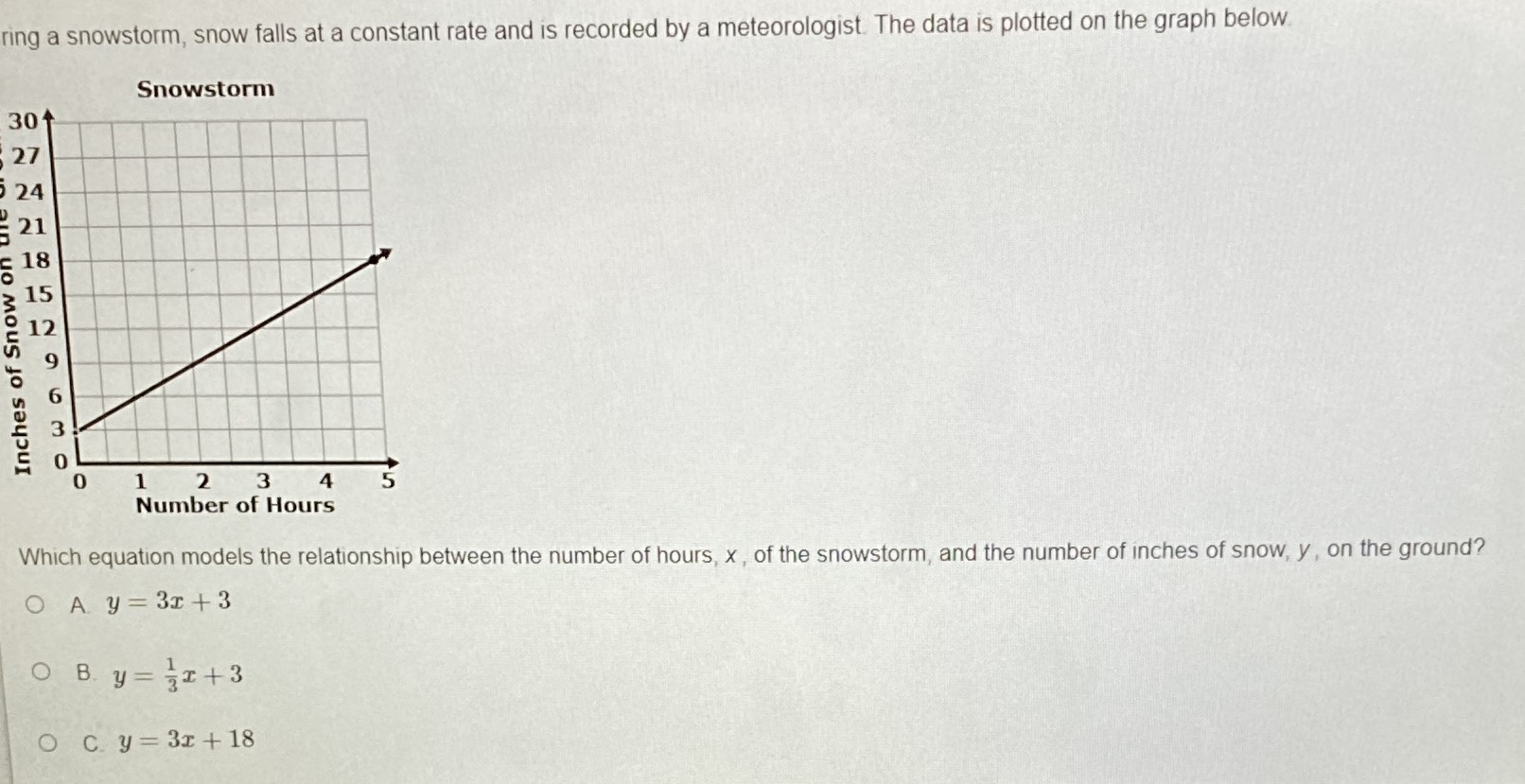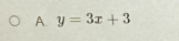### Still have math questions?

Algebra
Questionring a snowstorm, snow falls at a constant rate and is recorded by a meteorologist. The data is plotted on the graph below.

Which equation models the relationship between the number of hours, $$x$$ , of the snowstorm, and the number of inches of snow, $$y ,$$ on the ground?

A. $$y = 3 x + 3$$

B. $$y = \frac { 1 } { 3 } x + 3$$

C. $$y = 3 x + 18$$